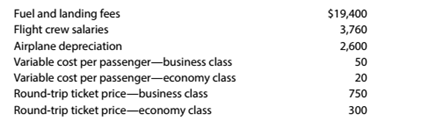Chapter 11, Problem 11.21E

Chapter
Section
Textbook Problem

Break-even sales and sales mix for a service company Yellow Dove Airways provides air transportation services between Portland and Minneapolis. A single Portland to Minneapolis round-trip flight has the following operating statistics:It is assumed that the fuel and landing fees, crew salaries, and airplane depreciation are fixed, regardless of the number of seats sold for the round-trip flight. a.Compute the break-even number of seats sold on a single round-trip flight for the overall product. Assume that the overall product is 10% business class and 90% economy class tickets. b.How many business class and economy class seats would as sold at the break-even point?

To determine

Concept Introduction:

Cost Volume Profit (CVP) Analysis:

The Cost Volume Profit analysis is the analysis of the relation between cost, volume, and profit of a product. It analyzes the cost and profits at the different level of production, in order to determine the breakeven point and required the level of sales to earn the desired profit.

Contribution margin means the margin that is left with the company after recovering variable cost out of revenue earned by selling smart phones. The formula for contribution margin is as follows:

Contribution margin = Sales - Variable cost.

Similarly contribution margin ratio = Contribution/sales

Breakeven Point:

The Breakeven point is the level of sales at which the net profit is nil. It can be explained as a situation where the business is generating a sale that is equal to the expenses incurred and hence no profits no loss. Breakeven point in units is calculated with the help of following formula:

Breakeven point (units) = Total Fixed Costs(Sales Price Per unit -Variable Cost per unit)

Weighted Average Contribution Margin:

Weighted Average Contribution Margin is calculated for two products with the help of following formula:

Weighted Average Contribution Margin = (Contribution margin of Product A * Weight of product A)                                                                       + (Contribution margin of Product B * Weight of product B)

Requirement-a:

To Calculate:

The Overall Breakeven Sales unit

Explanation

The Overall Breakeven point in units is calculated as follows:

 Business Economy Overall Unit Selling Price (A) $750$ 300 Unit Variable Cost (B) $50$ 20 Unit Contribution Margin (C) =(A-B) $700$ 280
To determine

Concept Introduction:

Cost Volume Profit (CVP) Analysis:

The Cost Volume Profit analysis is the analysis of the relation between cost, volume, and profit of a product. It analyzes the cost and profits at the different level of production, in order to determine the breakeven point and required the level of sales to earn the desired profit.

Contribution margin means the margin that is left with the company after recovering variable cost out of revenue earned by selling smart phones. The formula for contribution margin is as follows:

Contribution margin = Sales - Variable cost.

Similarly contribution margin ratio = Contribution/sales

Breakeven Point:

The Breakeven point is the level of sales at which the net profit is nil. It can be explained as a situation where the business is generating a sale that is equal to the expenses incurred and hence no profits no loss. Breakeven point in units is calculated with the help of following formula:

Breakeven point (units) = Total Fixed Costs(Sales Price Per unit -Variable Cost per unit)

Weighted Average Contribution Margin:

Weighted Average Contribution Margin is calculated for two products with the help of following formula:

Weighted Average Contribution Margin = (Contribution margin of Product A * Weight of product A)                                                                       + (Contribution margin of Product B * Weight of product B)

Requirement-b:

To Calculate:

The Breakeven Sales unit for each product

Still sussing out bartleby?

Check out a sample textbook solution.

See a sample solution

The Solution to Your Study Problems

Bartleby provides explanations to thousands of textbook problems written by our experts, many with advanced degrees!

Get Started

Find more solutions based on key concepts